# Elegy for a Dying Field: Comments on “Detection of Direct Sequence Spread Spectrum Signals Based on Deep Learning,” by F. Wei et al

Black-box thinking is degrading our ability to connect effects to causes.

I’m learning, slowly because I’m stubborn and (I know it is hard to believe) optimistic, that there is no bottom. Signal processing and communications theory and practice are being steadily degraded in the world’s best (and worst of course) peer-reviewed journals.

I saw the accepted paper in the post title (The Literature [R177]) and thought this could be better than most of the machine-learning modulation-recognition papers I’ve reviewed. It takes a little more effort to properly understand and generate direct-sequence spread-spectrum (DSSS) signals, and the authors will likely focus on the practical case where the inband SNR is low. Plus there are lots of connections to CSP. But no. Let’s take a look.

The authors start off by providing context for the use of DSSS signals in terms of covert signaling, or ‘concealment,’Figure 1. A good start–DSSS signals are indeed often used for covert signaling. This is one of the reasons they are often encountered in the wild with low inband SNR.

This is an encouraging start. When reviewing possible methods of detecting such weak DSSS signals, the authors do mention CSP (barely), but then sort of just leaving it hanging there. They admit that the papers they cite show that the CSP methods can outperform conventional methods (energy detection), but then, that’s it. Tip-toe quietly away…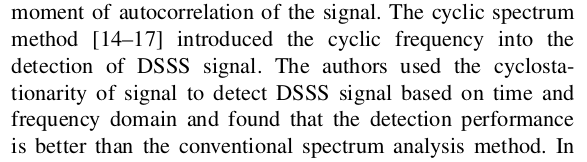Figure 2. The only mention of cyclostationarity in the paper. After mentioning that the cyclic methods give good performance, the authors ignore it and focus on stationary-signal parameters such as the autocorrelation. Why?

OK, fine. This literature review is followed by the obligatory claims that neural networks are inherently superior, because of reasons. Check out the logic in Figures 3 and 4. Neural networks can find hidden stuff (what, exactly, hidden stuff? No one knows.) Professionals can find feature stuff. Therefore neural networks can be expected to outperform the features. Uh, why is it not possible for the professionals to find optimal features, whether or not they are hidden? Is math that broken?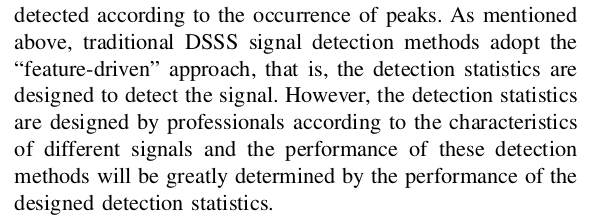Figure 3. ‘Detection statistics are designed by professionals.’ They probably mean ‘detection features.’ In any case, this is the usual dig at either the poverty of mathematics or the distasteful association of a human with an algorithm. I guess. Note, however, that the endless trial-and-error involved in creating all the layers and parameters of a modern neural network is never denigrated as being designed by professionals (ick).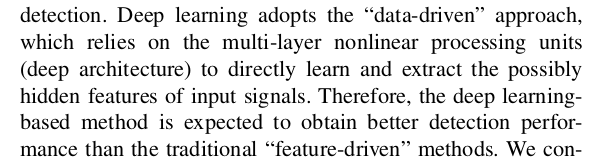Figure 4. A form of logic I can’t grasp. Premise: Deep learning extracts possibly hidden features. Conclusion: Deep learning is therefore better than traditional feature-driven methods. Why? Why can’t the traditional features match (equal) the extracted possibly hidden features? There is a missing premise or two: “hidden is better than traditional,” or “traditional can’t possibly find hidden.” Which is amusing since a common way of explaining CSP is through the notion of “hidden periodicity.”

Then we start to get technical. We quickly encounter the statement that the autocorrelation function for DSSS signals is periodic–see Figure 5. It is not. Except! Except in the special case in which the information symbols driving the DSSS modulator are periodic. In which case the signal is no longer ‘concealed’ in the noise, since it is periodic and therefore has only impulsive spectral components. And it is no longer a communication signal.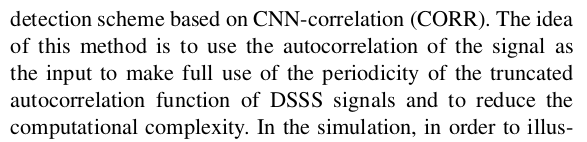Figure 5. Claim that the autocorrelation of a DSSS signal is periodic. It is not unless the information symbols driving the DSSS modulator are constant or, more generally, periodic themselves. Which they are not in normal settings. This is the first hint that the mathematical properties of the periodically repeated m-sequence (used as the spreading code) are conflated with the mathematical properties of the DSSS signal itself, a major error.

Moving on, in Figure 6 we find a mathematical expression for the DSSS signals considered in the work. These equations describe a rectangular-pulse DSSS BPSK (because$d_i$ is binary) signal, which is impractical, but OK. (Later we see that they don’t ever use this impractical signal. They use a square-root raised-cosine DSSS BPSK signal. Which is great!)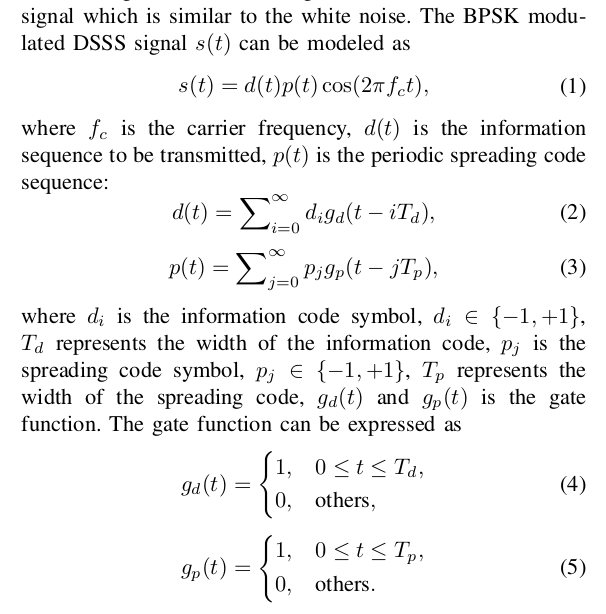Figure 6. The mathematical definition of DSSS provided in [R177]. This is a reasonable model for rectangular-pulse DSSS BPSK, provided the sequence is periodic. The authors state that is the periodic spreading code sequence, but it is actually a function of the real time variable . The code sequence is represented by .

Then things start to degrade. There appears the curious statement “Because the autocorrelation function of the DSSS signal is the product of the autocorrelation function of the signal code and the autocorrelation function of the pseudo-random code, it also has the correlation characteristics of the pseudo-random sequence to a certain extent.” They they go on to focus exclusively on the autocorrelation of the periodic spreading sequence or, more precisely, on the function$p(t)$ in (3), which is the pseudo-random sequence but where each point in the sequence is repaced by a rectangle of width$T_p$ (I will call this the ‘stretched’ sequence). See Figures 7 and 8.

But the statement in italics above is false in at least two ways. The autocorrelation function for a DSSS signal is not the product of the autocorrelation functions of (2) and (3). We know the autocorrelation function for the binary pulse-amplitude-modulated (PAM) signal in (2) is a triangle and we know the autocorrelation function of (3) is a periodic triangular pulse train. So the product would be the product of two different triangles centered at the origin. But that is not the autocorrelation. Instead, it is equal to the effective pulse correlated with itself, since the combined signal (2)-(3) can be interpreted as a binary PAM signal with an unusual pulse made up of one period of (3). We have explicit formulas for the cyclic cumulants and cyclic polyspectra for such signals, which feature the autocorrelation and power spectrum as special cases.

The second way the italicized statement above is incorrect is more fundamental, and therefore more depressing. Recasting the statement into simpler terms:$z(t) = x(t)y(t)$, therefore$z(t)$ has the properties, somewhat, of$x(t)$. But what if$y(t) \equiv 0$? What if$x(t)$ and$y(t)$ don’t even have any common support over the real numbers$t$? It is quite simple for$z(t)$ to not have any of the properties of$x(t)$. In the present case,$y(t)$ is periodic, and$x(t)$ is an energy signal with support highly concentrated at the origin. So$z(t)$ isn’t anything like$y(t)$. Better said, the autocorrelation function for the DSSS signal isn’t anything like the autocorrelation for the stretched periodic sequence$p(t)$. Let’s adduce some evidence in an interlude!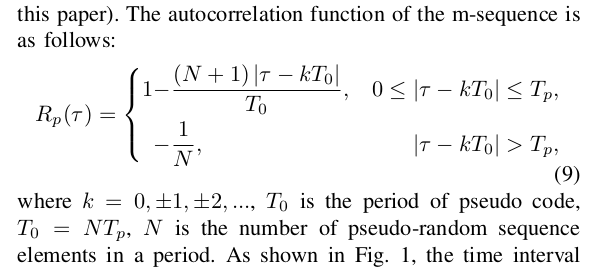Figure 7. The authors’ attempt at expressing the autocorrelation of the periodic m-sequence. Note that this cannot be the autocorrelation of the m-sequence because it explicitly includes the chip-pulse with . It is closer to the autocorrelation function of (3), but as expressed, it isn’t even a function. For example, for and , you get . But for and you get .Figure 8. A graphical representation of the function in the authors’ (9) (Figure 7 here). The lower plot is actually OK for the autocorrelation of the periodic function (3).

### Interlude: The Real Deal on the Autocorrelation for DSSS Signals

Figures A-C show the estimated autocorrelation functions for noiseless simulated rectangular-pulse and SRRC-pulse DSSS BPSK signals. Of particular importance to the paper is the fact that the autocorrelation functions are negligible (theoretically zero) for values of the lag variable$\tau$ exceeding$NT_p = NT_{chip}$ samples. This is due to the basic formula for the autocorrelation of pulse-amplitude-modulated signals and the fact that the PAM representation of the DSSS signal has a pulse width that is$NT_p$ samples long (exact for rectangular, approximate for SRRC).

Figures D-F show the corresponding power spectrum estimates.Figure A. Estimated autocorrelation functions for rectangular-pulse DSSS BPSK with various spreading-sequence lengths and eight samples per chip (as in the paper). Note that for the case of interest to the authors of [R177], the autocorrelation is negligible for .Figure B. Estimated autocorrelation functions for SRRC-pulse DSSS BPSK with various spreading-sequence lengths and eight samples per chip (as in the paper). Note that for the case of interest to the authors of [R177], the autocorrelation is negligible for . The prominent peak is at .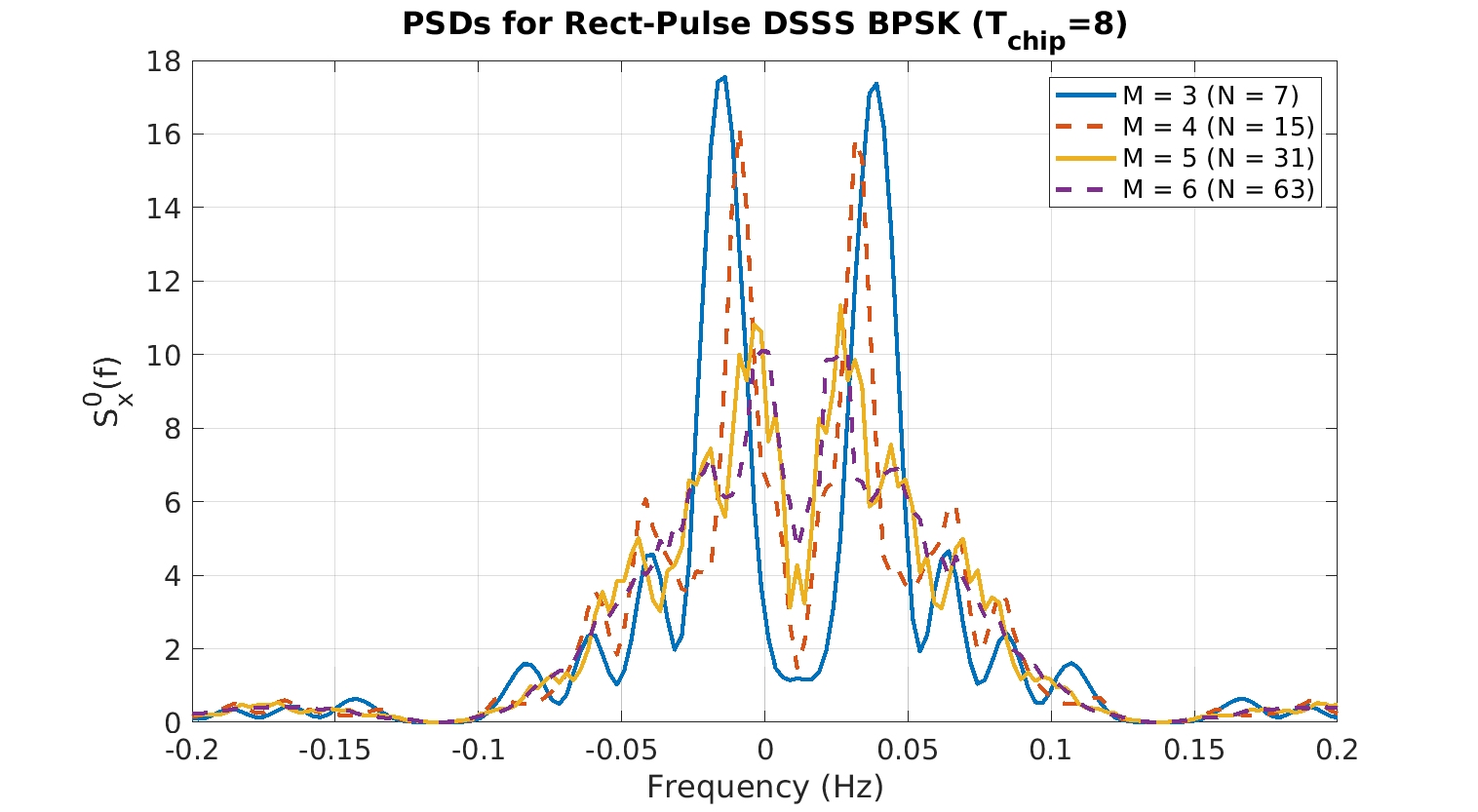Figure D. Estimated power spectra for rectangular-pulse DSSS BPSK corresponding to the cases of Figure A. Just for fun.Figure E. Estimated power spectra for SRRC-pulse DSSS BPSK for the cases in Figure B. Just for even more fun.Figure F. Estimated power spectra for the stretched periodic MLSR sequence (3) corresponding to the cases in Figure C. Note here that the stretched signal is periodic, so that the power spectra contain only impulsive components, which are smoothed into rectangles here due to the use of the FSM.

### End of Interlude: Back to the Paper

The authors then present something they characterize as a ‘traditional autocorrelation-based DSSS detector,’ but which is really just a mixed-up detection scheme based on the erroneous idea that the autocorrelation for DSSS is periodic. So we see in Figures 9-10 this idea that you have to look in different$\tau$ intervals of the autocorrelation function to gather up the peaks, as if they were operating on, say, Figure C instead of Figure A or B.

In Figures 11-12, we see that the authors want to truncate the autocorrelation that is used as a neural-network input so that the complexity of the inference is reduced (and training is easier too). Again we see this idea that the DSSS autocorrelation function has a peak in “the PN code period.” In reality, it has a dominant peak at$\tau=0$ representing the sum of the noise and signal powers, and lesser peaks out to$|\tau| < NT_p$.

When we get to the simulations, we see that the model (2)-(3), which is rectangular-pulse DSSS BPSK, is not actually used. Instead a more practical square-root raised-cosine DSSS BPSK signal is used (which is good!) and we now know that the value of$T_p = 8$ samples.Figure 13. Well, I guess (2) and (3) aren’t the signal model after all. However, it is good to see the authors use a more practical version of DSSS in their experiments.

In Figure 14 we learn that the network is trained using a single value of the code length (equivalently, a single value for the length of the shift register), and that length is$7$, which means the shift-register has length$3$. This is a very small spreading factor, not used in practice in the authors’ own context of ‘concealment.’ But OK.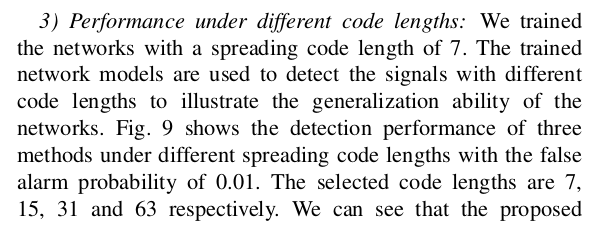Figure 14. So here we get the information about the length of the spreading sequence used to generate the DSSS signals in the training/testing dataset. It is 7, which is the length of the sequence, which means the M parameter in the shift-register is 3. Extremely low for ‘concealment’ purposes, which is the first thing the authors mention in this paper.

Finally, in Figure 15 we learn of an experiment involving the truncation of the autocorrelation estimate (see Figures 11-12). The authors then try to explain the appearance of the performance curves in their Figure 14, and so this is where we see the final effect of the conflation of the autocorrelations that I described above. The performance ordering is supposed to arise due to the key autocorrelation lag of$\tau = 56$, which is equal to a single code period since$N=7$ and$T_p = 8$, and$7\times 8 = 56$. And indeed, the autocorrelation of the stretched periodic spreading sequence does have a peak at$\tau = 56$ (Figure C). But the autocorrelation of the DSSS signal does not–it is negligible for that$\tau$!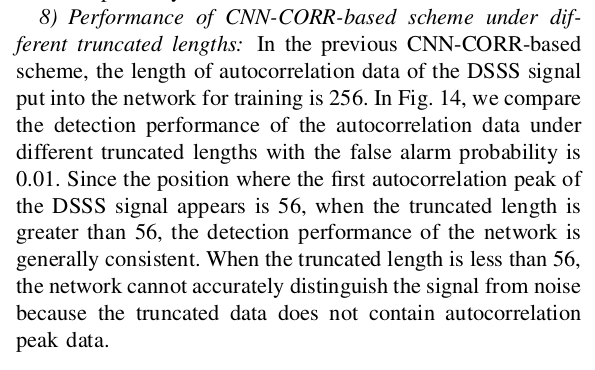Figure 15. An explanation of the cause of the ordering of the performance curves in the authors’ Figure 14. The answer is ’56’ because that is the autocorrelation lag for which the first recurring peak in the DSSS autocorrelation appears. (This is not correct. Not even close. Not even wrong?)

### Conclusion and Update to The New Reviewing Scheme

The mathematical errors–both elementary and in probability–are egregious. So what, you may say, same as ever. But this is the IEEE Transactions. The greatest of the holies. This particular Transactions journal is just a rung (maybe two?) below Transactions on Signal Processing. How can this happen?

I don’t put much of the blame on the authors. We all make mistakes, we are all learning and trying to do something important and novel. I blame the editors and reviewers. How could the reviewers miss these key errors, errors that throw all the authors’ conclusions into serious doubt?

The reason both the authors and the reviewers miss the mathematical errors that invalidate the comparison of the methods based on the autocorrelation function, and which cast serious doubt on the signal model, is that the cult(ure) of machine learning has obscured the feedback connection between effect and cause.

I generate or obtain some data. I train my machine. I test my machine. I plot the performance. Q: Why is the performance this way? A: Shrug. That’s what the machine did. Our increasing acceptance of this mental dynamic (by me too) is what leads to the kinds of errors we see in this paper, and in many others, as I continue to document. In the present paper, we get the magical explanation of ’56’ as the reason for some performance graph’s appearance. Is that really the cause of that effect? One way to be sure is to delve into that answer–plot the autocorrelation function of the input DSSS signals. Are they periodic as stated? No. Why not? Maybe the signal model or generation is wrong–better check the literature. No. Is there at least a peak at 56? No. Why bother doing any of those checks, though, if the reviewers don’t care? Either the reviewers are true ML believers and also don’t care about the math, cause, and effect, or they are simply incompetent. Either way, it doesn’t pay the authors to get it right by performing various cross-checks. What pays is lengthy descriptions of the layers of the machine and Tables like Table I–shibboleths. That’s what the reviewers are looking for, not cause and effect, or mathematical fidelity. I think. Would like to know for sure. In any case, graduate students and researchers new to the field suffer. And everyone continues to get the impression that ML is triumphing.

Because I’d like to know for sure what reviewers are paying attention to, I add this rule to the New Rules of Reviewing I proposed in the latest correntropy debacle:

All reviews become public after a paper is published.

Let’s see what’s really going on in reviews of papers like [R177].

### Post Script